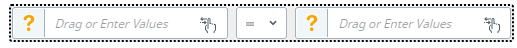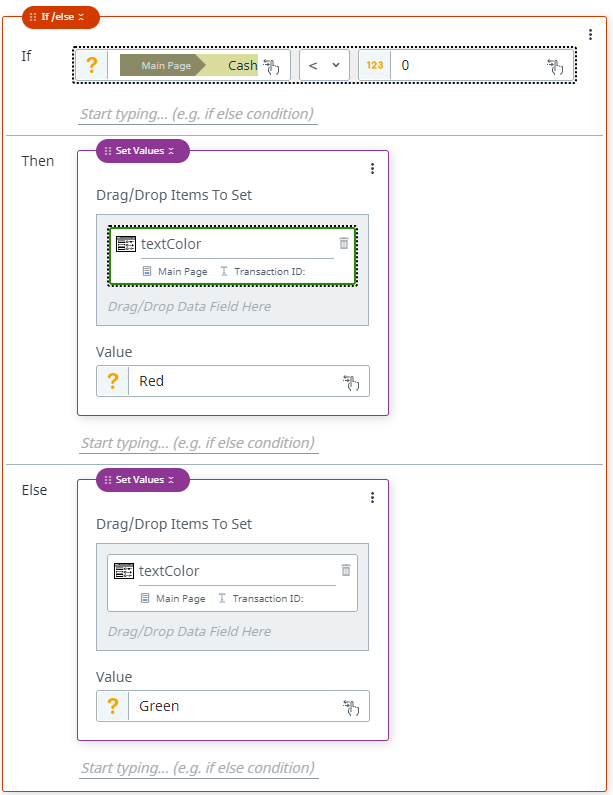# Rule: If-Then-Else

Provides support for conditional statements

We often want to "conditionally" do things in our app, for example if the value of a label is <0, we want it to be red. We can do things conditionally in our app using the if-then-else statement combined with conditional expressions.

In Apps Studio, the If-Then-Else rule allows you to specify:

1. the condition(s) to check (that's goes in the If statement)
2. what happens if the condition is true (that's goes in the Then statement)
3. what happens if the condition is false (that's goes in the Else statement)

# The If statement

There are two kinds of conditional statements that can be used inside the If:

• Compare: Used to compare two values.
• Group: Used to group multiple comparisons (And/Or).

## Compare

The compare function allows you to write a conditional expression - something that evaluates to true or false.Each condition specifies two values to compare and a comparison operation.

These values can be:

• a reference to a control property
• a static value (i.e. a number or a string)

The comparison operation must be one of the following:

Comparison operation

Symbol

Description

Example

Less than

<

Returns true if the left value is less than the right value

3 < 6

Less than or equal

Returns true if the left value is less than or equal to the right value

Cash In Value ≤ 0

Greater than

Returns true if the left value is greater than the right value

5 > 2

Greater than or equal

Returns true if the left value is greater than or equal to the right value

Cash Out Value ≥ 0

Equal

=

Returns true if the left value is equal to the right value

State = WA

Not Equal

Returns true if the left value is not equal to the right value

Transaction Id ≠ 0

## Group

The Group function allows you to combine multiple conditional expression together. This allows us to make more complex conditional expressions like "if both X and Y are true" or "if either X or Y are true" in our apps.

# The Then/Else Statement

The Then and Else statements can be any rule, meaning you can nest statements.

# Example

Scenario: When the "Submit" button is clicked, a process runs and returns a transaction ID. The text color of the "Transaction ID" label should be red if the deposit is less than 0, otherwise it should be green.The text color of the "Transaction ID" label should be red if its value is less than 0, otherwise it should be green.Updated 26 days ago

# Rule: If-Then-Else

Provides support for conditional statements

### Suggested Edits are limited on API Reference Pages

You can only suggest edits to Markdown body content, but not to the API spec.